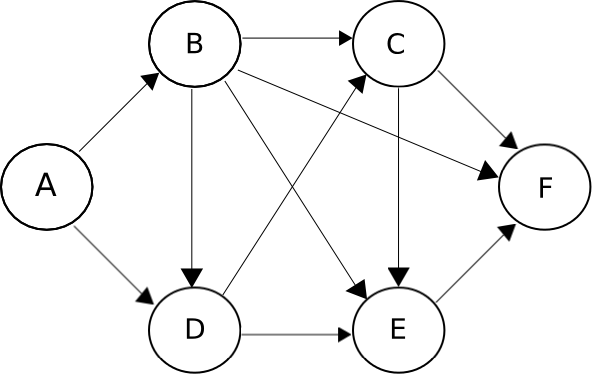Question 49

# The figure below shows the network connecting cities A, B, C, D, E and F. The arrows indicate permissible direction of travel. What is the number of distinct paths from A to F?Solution

The distinct paths are:

A -> D -> C -> F
A -> D -> E -> F
A -> D -> C -> E -> F
A -> B -> D -> C -> F
A -> B -> D -> E -> F
A -> B -> D -> C -> E -> F
A -> B -> C -> F
A -> B -> E -> F
A -> B -> F
A -> B -> C -> E -> F

So, the total number of distinct paths is 10